# Printable Fraction Decimal Percent ChartUpdated on Jan 28, 2022By Printablee Team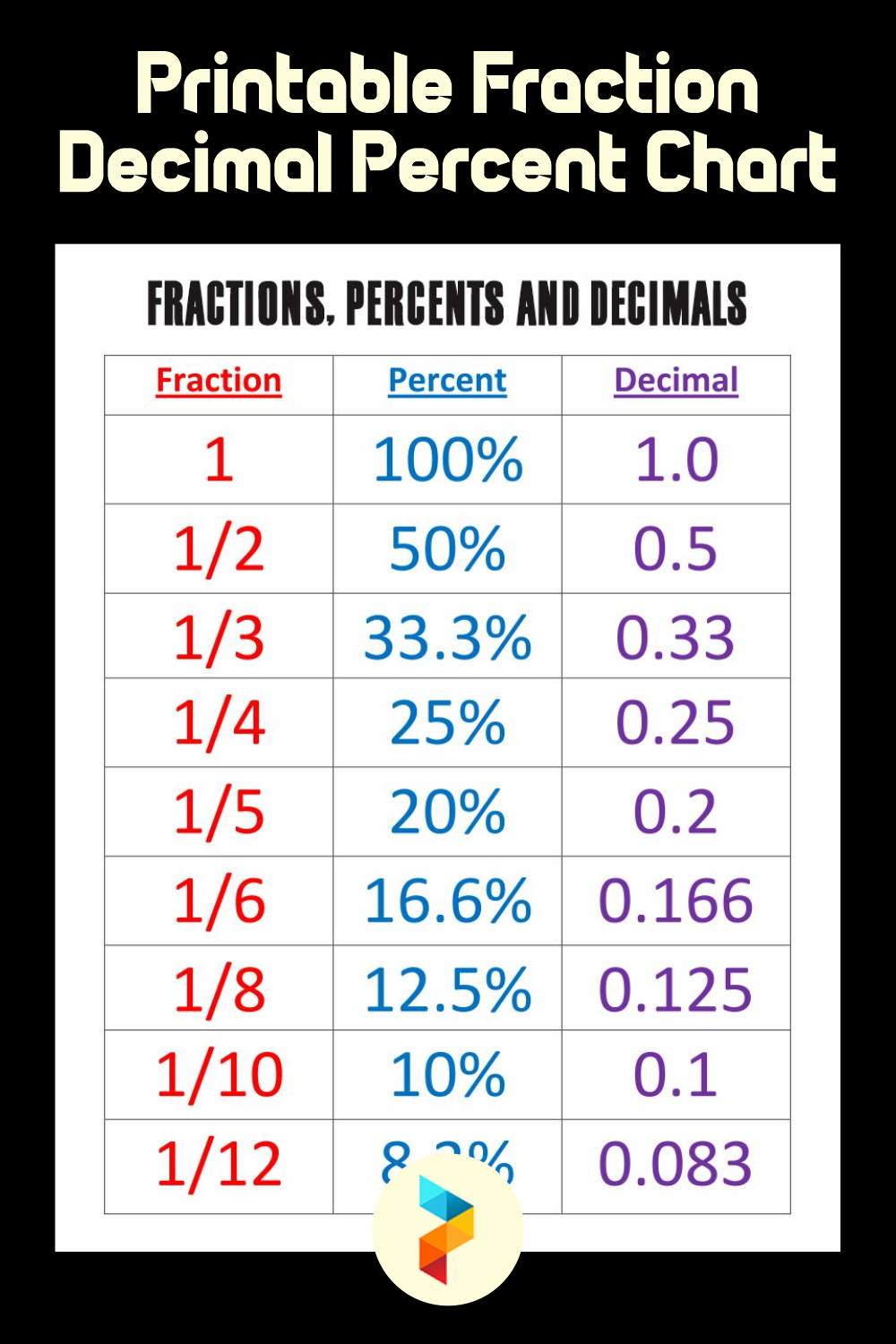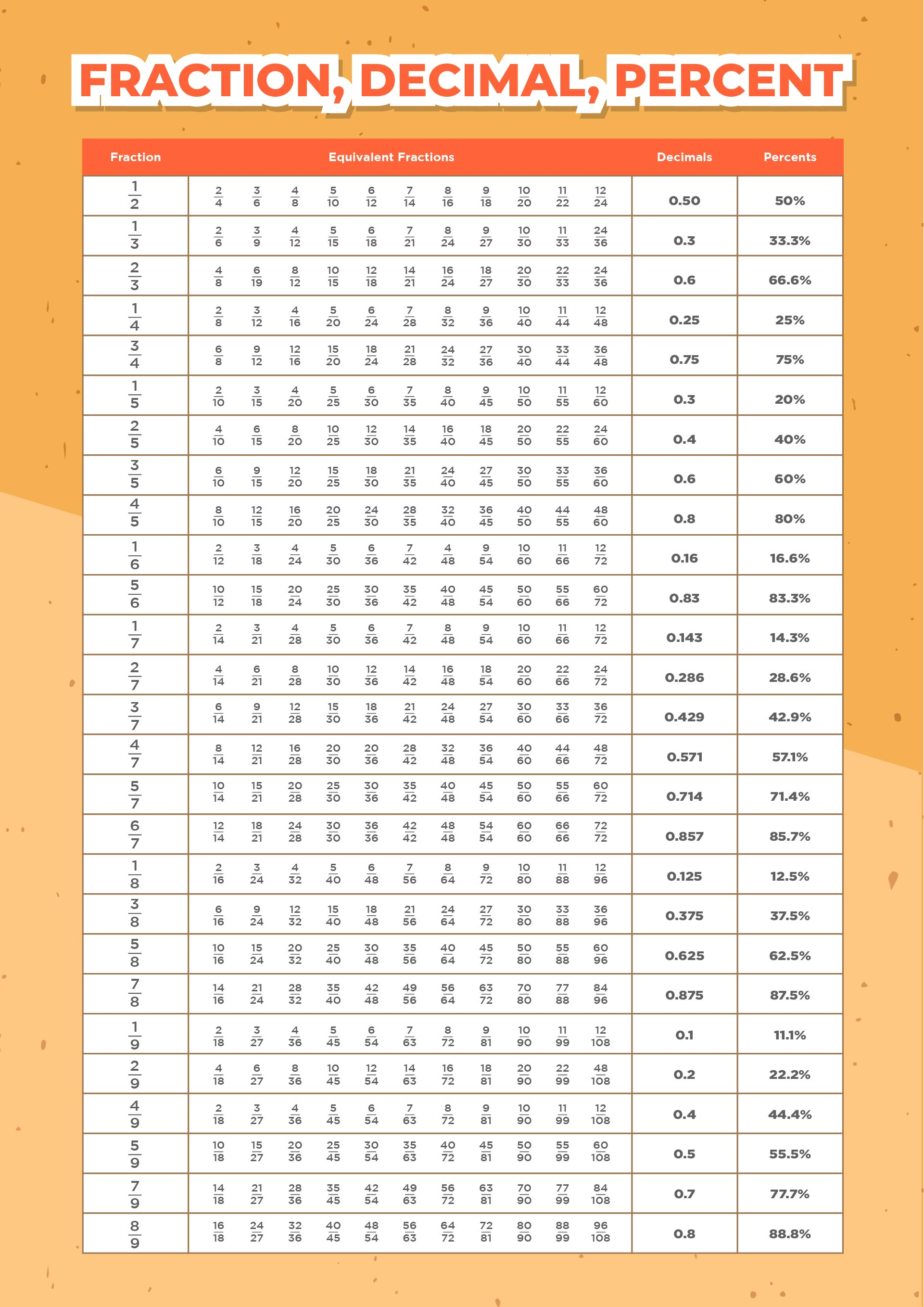### What is fraction decimal and percentage?

There are three ways to express fraction numbers or fractional numbers, namely ordinary fractions (fraction), decimal fractions (decimal), and percent numbers. Ordinary fractions can be translated into decimal fractions and percent numbers. I know it can be a little difficult to imagine for an elementary schooler, so first, we will give a common fraction decimal percentage chart to make it easier for kids to learn.

Ordinary Fractions – Ordinary fraction numbers or regular fraction numbers are numbers that show the comparison in the form a / b (pronounced a / b) in this case called a numerator and b is called a divisor. An example of a regular fraction is 1/3 (1 is the numerator and 3 is the denominator)

Decimal Fraction Numbers – A decimal fraction number or a decimal fraction number is a fraction number in the form of tenths, percentages, thousandths, tenths of thousands, and so on.

Percent Numbers – Percent number or percent number is the number in the form per hundred. The percent number is written as a number followed by the % sign (symbol). The figure 15% reads 15 percent means 15 percent.

I know it can be a little difficult for an elementary schooler, so first, we will give a common fraction decimal percentage chart to make it easier for kids to learn and memorize.

 Fraction Decimal Percent Chart Fraction Decimal Percent 1/2 0.5 50% 1/3 0.333… 33.333…% 2/3 0.666… 66.666…% 1/4 0.25 25% 3/4 0.75 75% 1/5 0.2 20% 2/5 0.4 40% 3/5 0.6 60% 4/5 0.8 80% 1/6 0.1666… 16.666…% 5/6 0.8333… 83.333…% 1/8 0.125 12.5% 3/8 0.375 37.5% 5/8 0.625 62.5% 7/8 0.875 87.5% 1/9 0.111… 11.111…% 2/9 0.222… 22.222…% 4/9 0.444… 44.444…% 5/9 0.555… 55.555…% 7/9 0.777… 77.777…% 8/9 0.888… 88.888…% 1/10 0.1 10% 1/12 0.08333… 8.333…% 1/16 0.0625 6.25% 1/32 0.03125 3.125% 1/100 0.01 1% 1/20 0.05 5% 1/10 0.1 10% 1/8 0.125 12½% 1/5 0.2 20% 1/4 0.25 25% 331/ 0.333... 3% 1/2 0.5 50% 3/4 0.75 75% 4/5 0.8 80% 9/10 0.9 90% 99/100 0.99 99% 1 100% 5/4 1.25 125% 3/2 1.5 150% 2 200%

### How do you write a decimal as a common fraction?

This will discuss how to convert a decimal number into a fraction.

Step 1 –  Write the decimal and divided by 1, for example: 0.25/1

Step 2 –  Multiply the top and bottom number by 10 for each number after the decimal point, (and if there are two digits after the decimal point, the number of zeros after the multiplier will also increase (100, 1000, etc.). For example : 0.25(x 100)/1(x100) = 25/100

Step 3 –  Reduce the fraction (simplify) with the biggest number that can divide it, for example: 25(:25)/100(:25) = ¼

Or you can just easily look up at the fraction decimal percentage chart that we’ve already provide.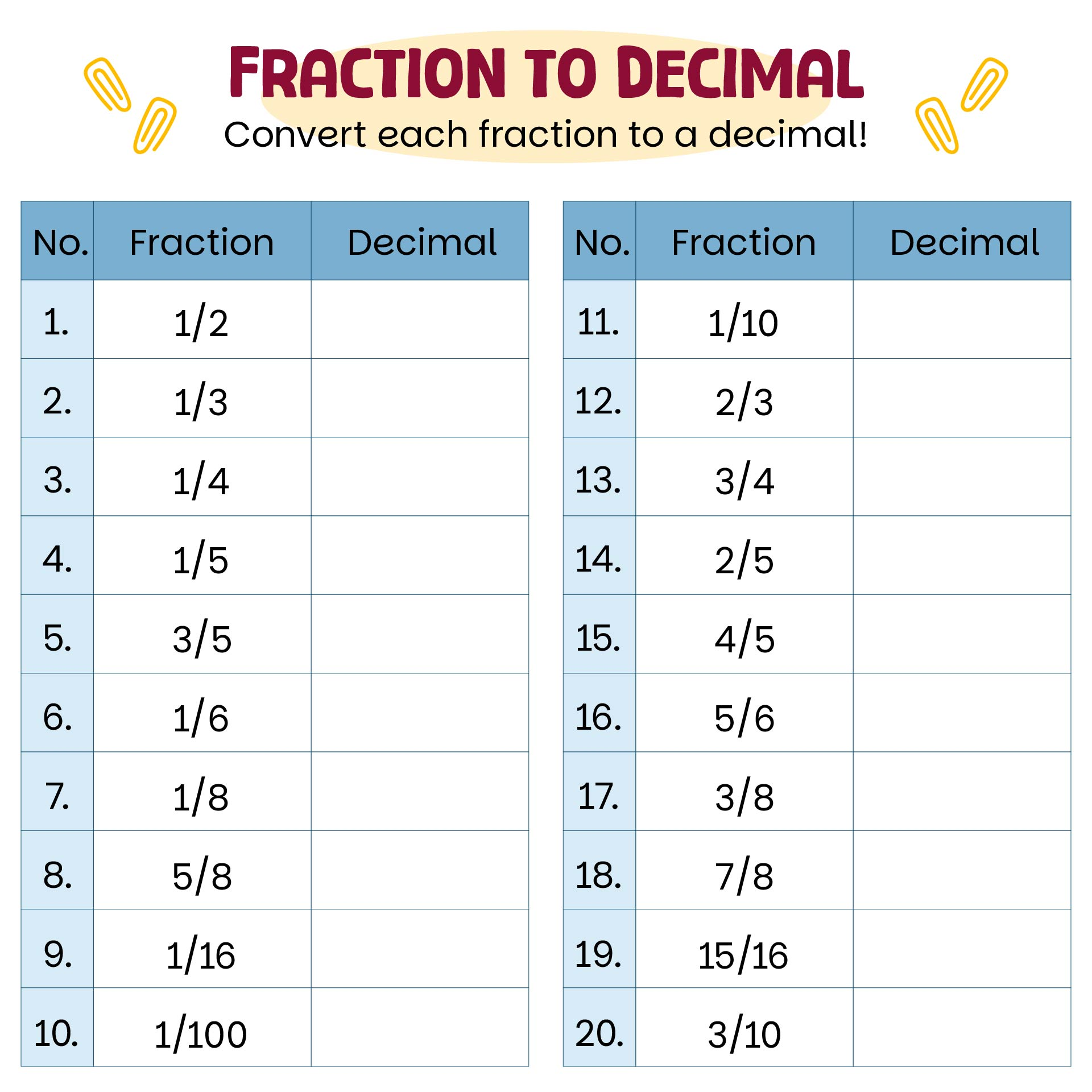We also have more printable chart you may like:
Decimal Equivalent Printable Chart
Printable Strip Fraction Chart
Decimal Chart Printable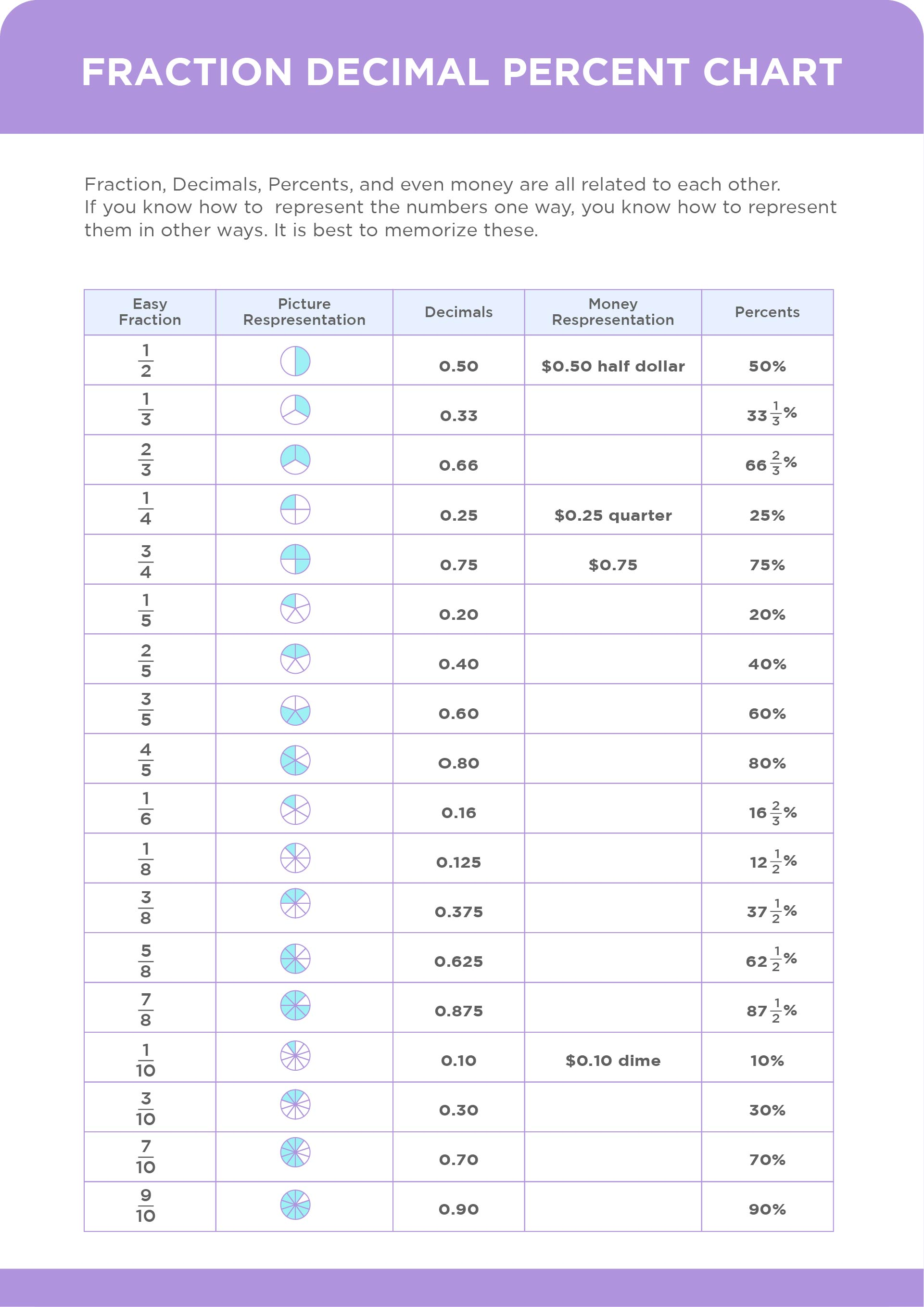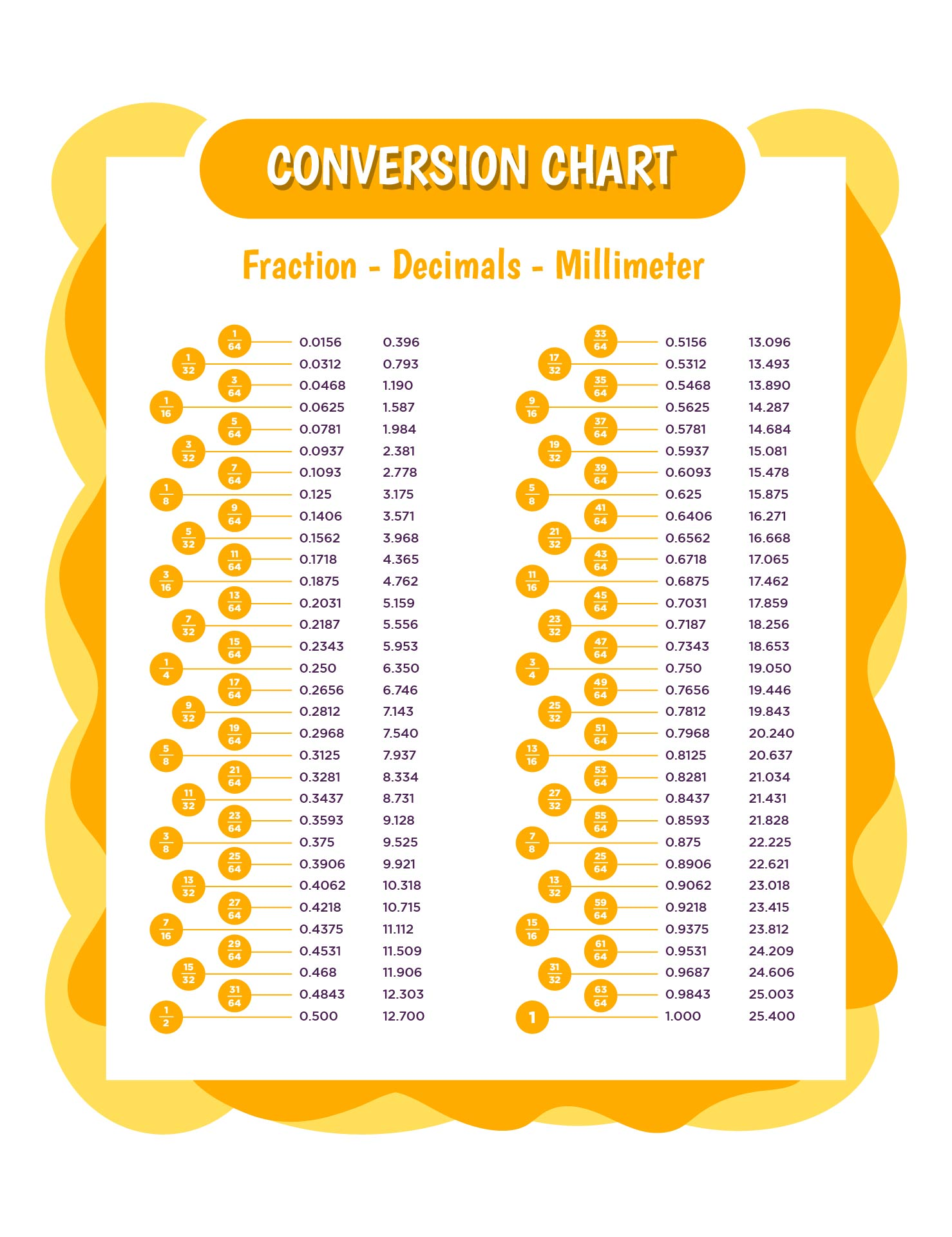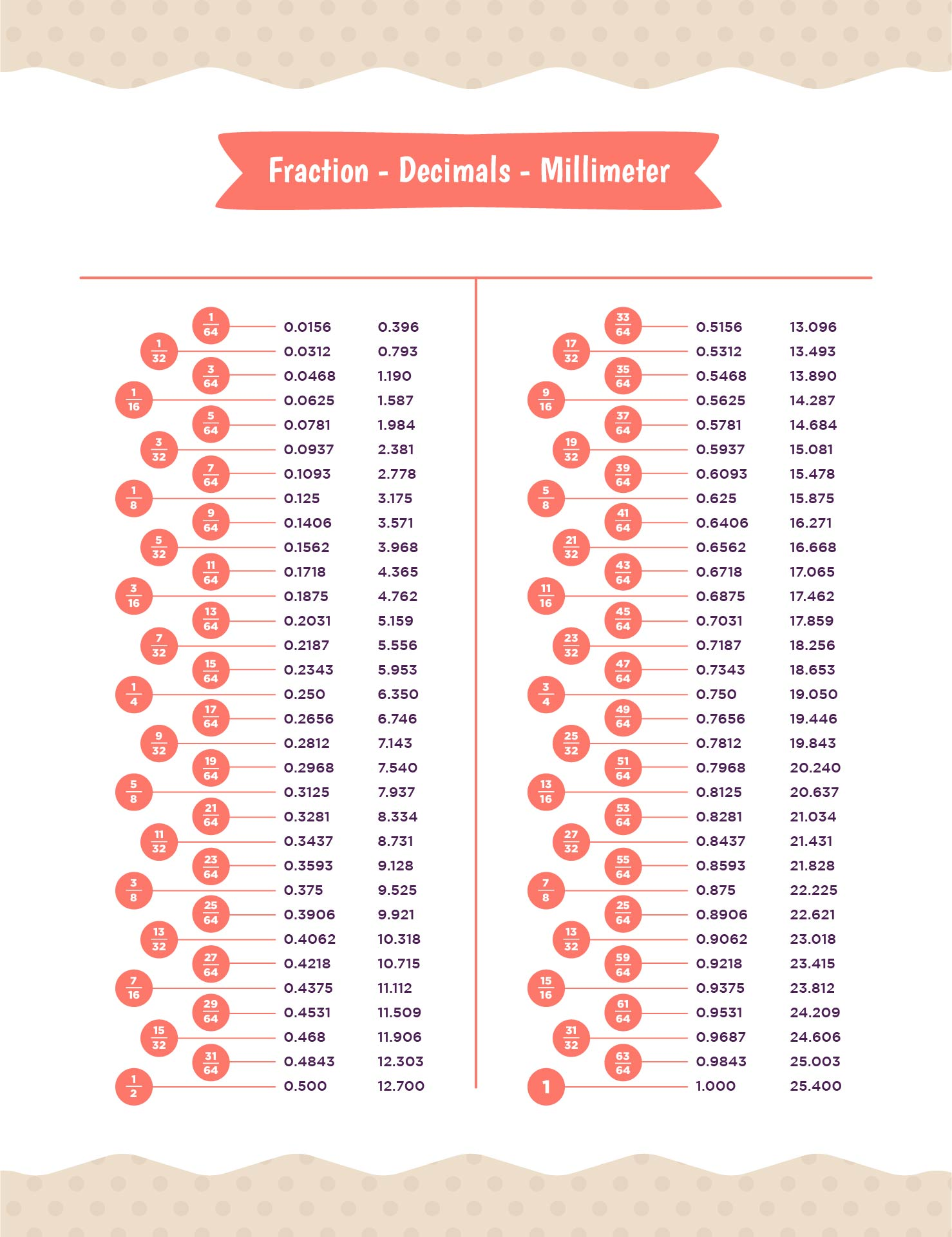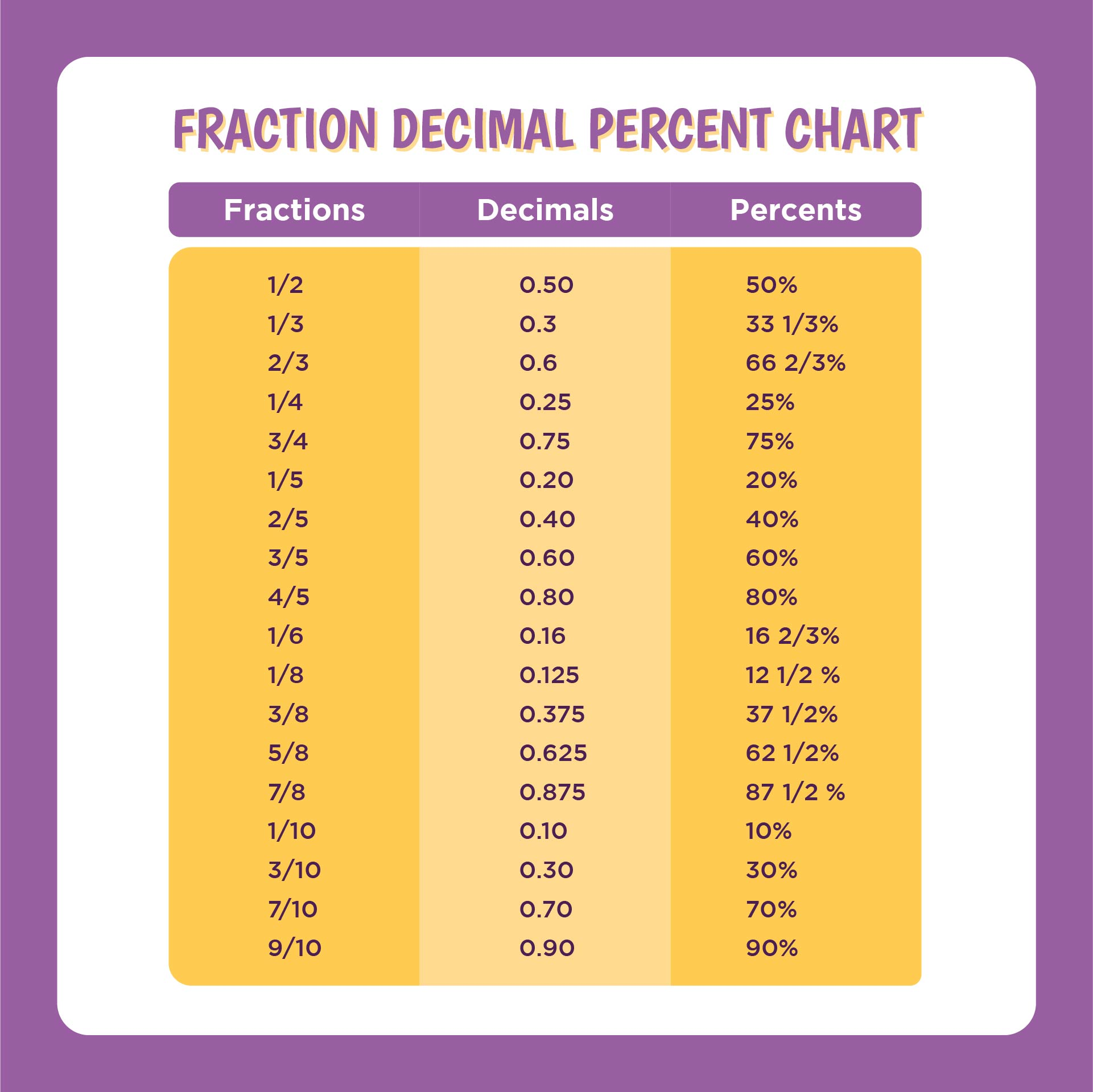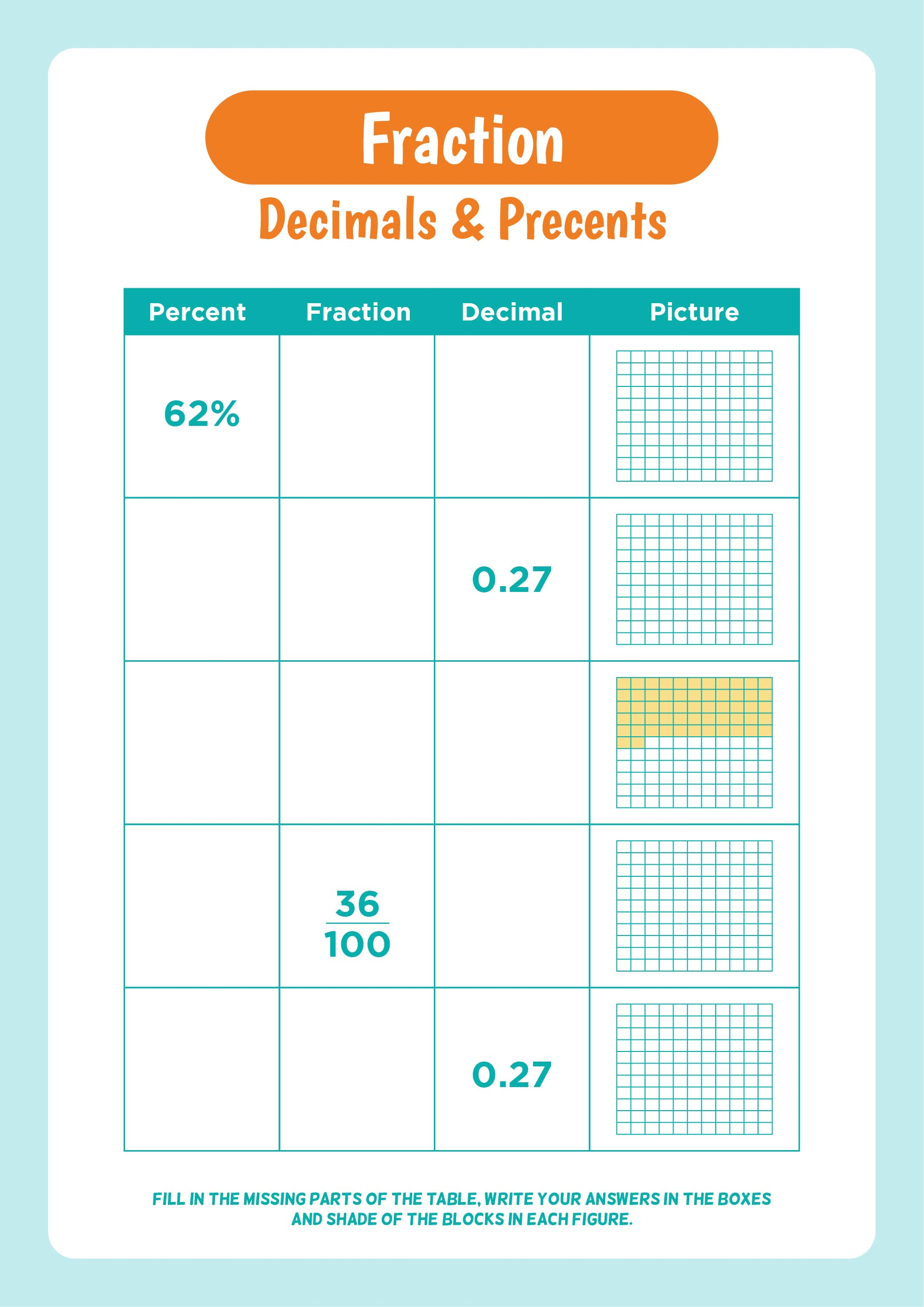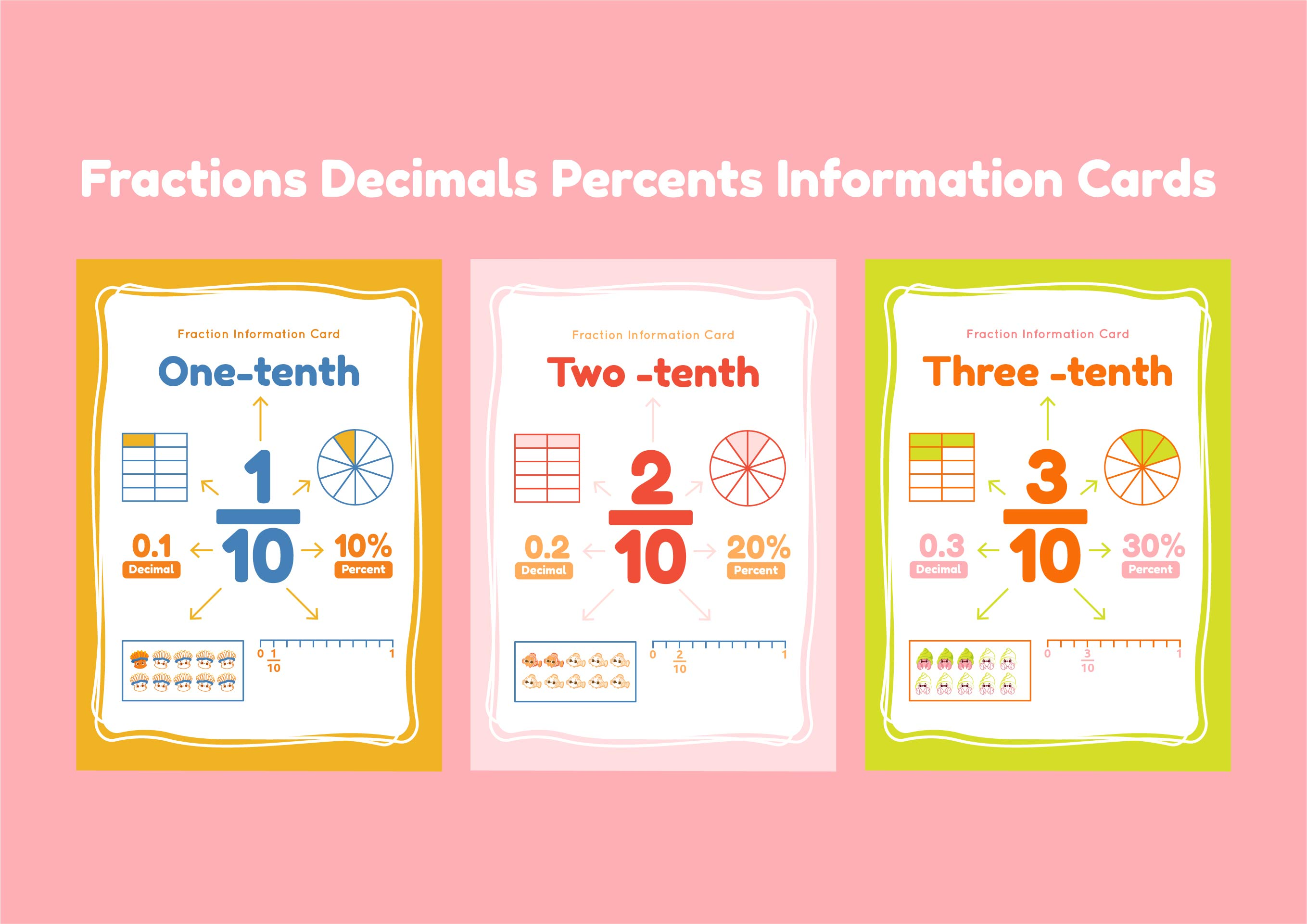### How do I write a fraction on a percentage basis?

This will discuss how to convert a fraction into a percent number, and it’s really easy.

Step 1 – Divide the top and bottom number, for example :  4/7 = 0,571

Step 2 – Multiply by 100%, for example : 0,571 x 100% = 57,1%

Or you can just easily look up at the fraction decimal percentage chart that we’ve already provide.